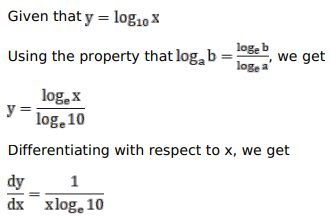# Solve this following

Question:

Mark $(\sqrt{ })$ against the correct answer in the following:

If $y=\log _{10} x$ then $\frac{d y}{d x}=?$

A. $\frac{1}{\mathrm{x}}$

B. $\frac{1}{\mathrm{x}}(\log 10)$

C. $\frac{1}{x(\log 10)}$

D. none of these

Solution: